Courses

# Test: Division - 1

## 10 Questions MCQ Test Mathematics (Maths) for Class 3 | Test: Division - 1

Description
This mock test of Test: Division - 1 for Class 3 helps you for every Class 3 entrance exam. This contains 10 Multiple Choice Questions for Class 3 Test: Division - 1 (mcq) to study with solutions a complete question bank. The solved questions answers in this Test: Division - 1 quiz give you a good mix of easy questions and tough questions. Class 3 students definitely take this Test: Division - 1 exercise for a better result in the exam. You can find other Test: Division - 1 extra questions, long questions & short questions for Class 3 on EduRev as well by searching above.
QUESTION: 1

### Pavani can use the fact 12 x 6 = 72 to help solve a related problem. Which of the following could be the problem she is trying to solve?

Solution:

12 × 6 = 72 This implies 72 ÷ 6 = 12 So, [ ] ÷ 6 = 12

QUESTION: 2

### 42 ÷ 7 = _______

Solution: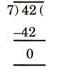QUESTION: 3

### Mr. Roy solved the division problem shown below on the blackboard.  252 ÷ 7 = 36  Which of the following could Mr. Roy use to check his answer?

Solution:

252 ÷ 7 = 36 This implies, 36 × 7 = 252

QUESTION: 4

Four baskets of apples are shown below.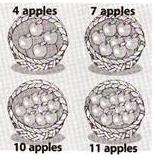Apples are moved from one basket to another until all the baskets contain the same number of apples. How many apples will there be in each basket in the last?

Solution:

Total number of apples
= 4+7+10+11 = 32
∴  Number of apples in each basket
= 32 ÷ 4 = 8

QUESTION: 5

Ms. Indira bought 8 packets of banana chips for Rs. 24. Which of the following number sentences could be used to determine the cost of one packet of banana chips?

Solution:

8 × [ ] = Rs.24
This implies
Rs.24 ÷ 8 = [ ]

QUESTION: 6

Suma arranged her toys in the pattern shown below.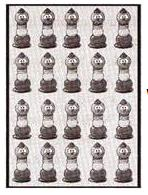What operation best shows how she arranged them?

Solution:

20 ÷ 5 = 4rows

QUESTION: 7

Ram saw 4 volleyball teams playing. Each team has 6 players, for a total of 24 players. Which number sentence is NOT in the same fact family as the others?

Solution:

2 × 4 = 8

QUESTION: 8

Ravi wants to show 15 pictures he took. He wants to put an equal number of pictures on 3 posters.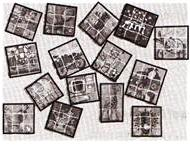Which number sentence shows how many pictures Ravi should put on each poster?

Solution:

15 ÷ 3 = 5

QUESTION: 9

Which number is missing in the number sentence?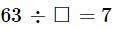Solution:

9 × 7 = 63
This implies
63 ÷  = 7

QUESTION: 10

There were 30 cookies on a plate for 9 children. If each child ate the same number of whole cookies, how many whole cookies did each child eat?

Solution: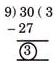So, each child ate 3 cookies